# Sphere

Ava calls her younger brother Jamie to show the picture of our planet Earth.

Ava asks, "Jamie, what do you see in the picture?"

Jamie undoubtedly nods his head and says, "It's our planet Earth."

"Great, Jamie!" says, Ava.

"Alright! Now tell me what the shape of our planet Earth is?"

Jamie says, "It's a circle."

Ava then says, "No Jamie. It's a sphere."

Jamie then argues that the Earth is a circle since it is round in shape.

"No, Jamie," says Ava, "a sphere is also round in shape, but a sphere is not the same as a circle."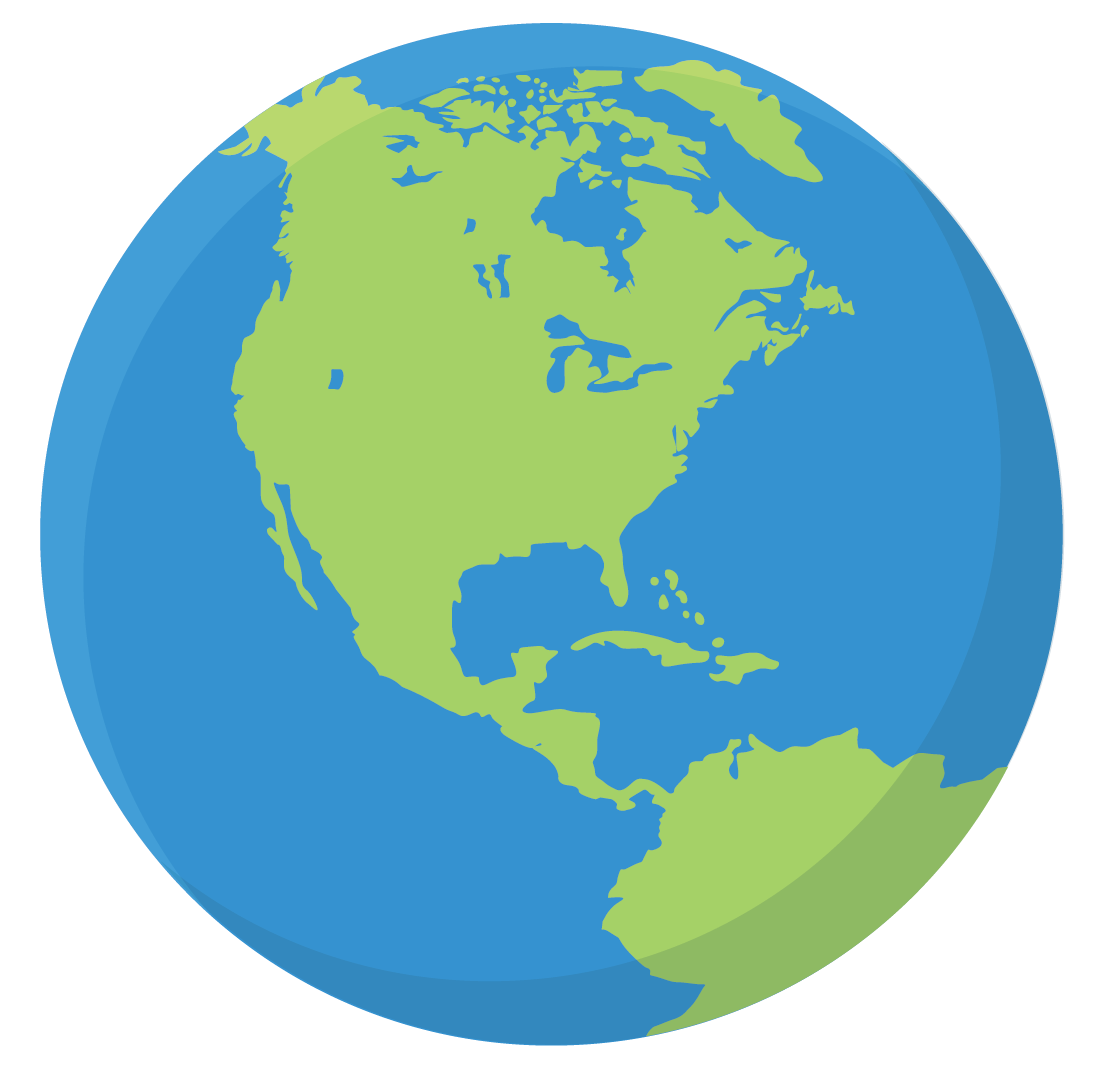Earth is a sphere!

## What Is a Sphere?

In geometry, a sphere is a three-dimensional solid figure, which is round in shape.

From a mathematical perspective, a sphere is a set of points connected with one common point at equal distances.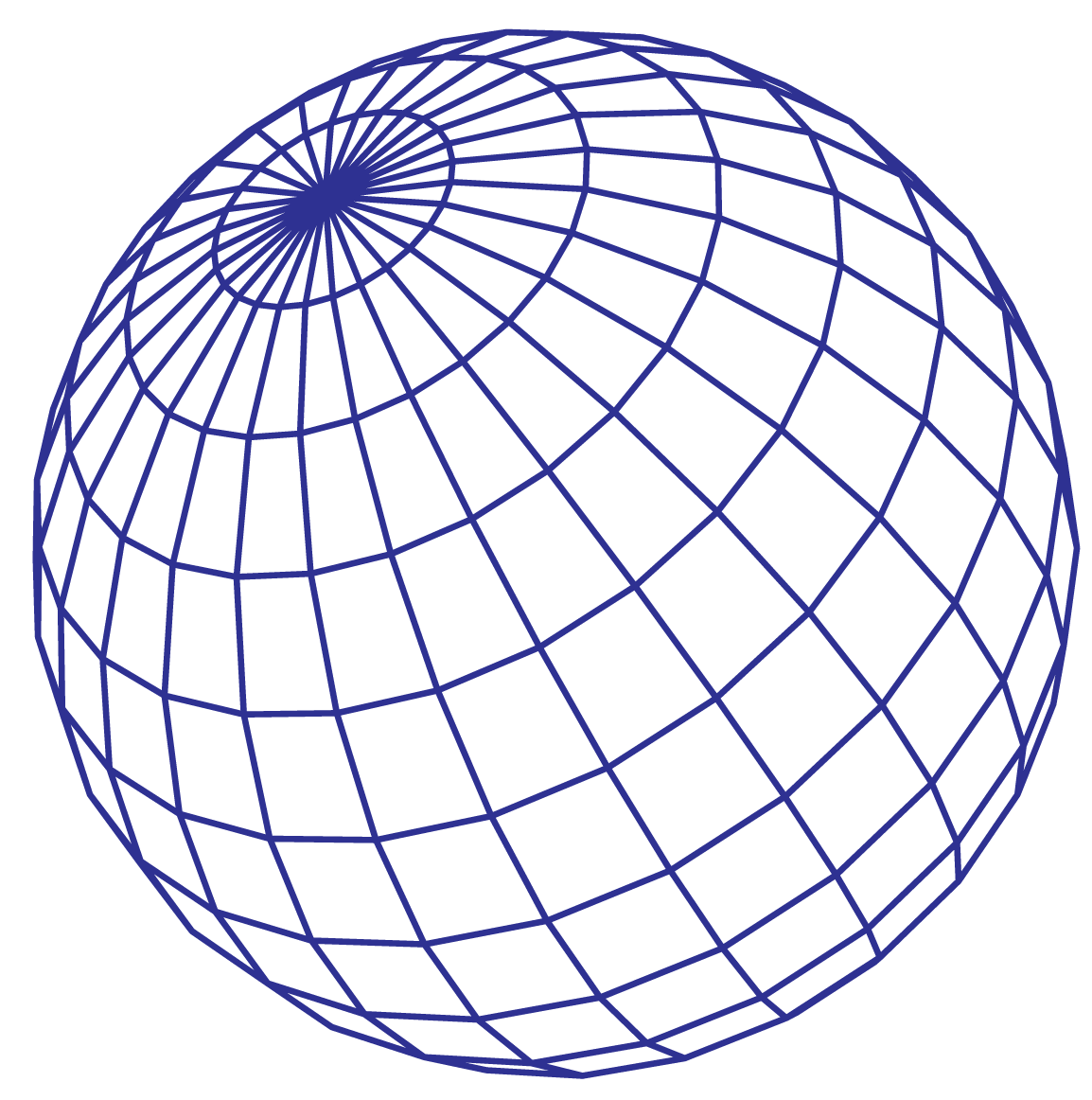Have you ever observed this shape around you?

Here are the images of a few objects shaped like a sphere.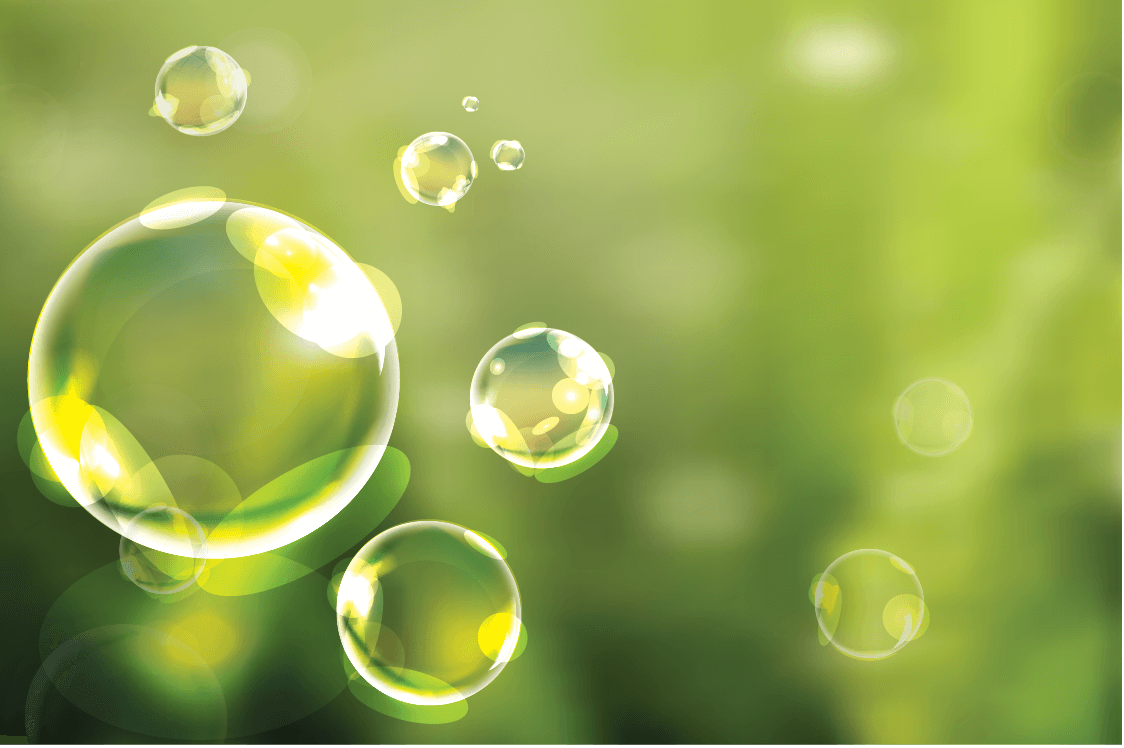## Formula of Area and Volume of a Sphere

### Formula For the Surface Area of a Sphere

Since the sphere is three-dimensional, we consider its surface area.

Therefore, the surface area of a sphere is obtained by the following formula.

 $$\begin{equation}\text{Surface Area of a Sphere} = 4\pi r^{2}\end{equation}$$

where r = radius of a circle.

### Formula For the Volume of a Sphere

The sphere volume is obtained by the following formula.

 $$\begin{equation}\text{Volume of a Sphere} = \dfrac{4}{3}\pi r^{3}\end{equation}$$

Now, you will be able to solve problems involving the sphere volume formula and sphere surface area.

## Properties of a Sphere

1. A sphere is symmetrical from all directions.

2. A sphere has only a curved surface area.

3. A sphere has no edges or vertices.

4. All the surface points of the sphere are at an equal distance from the center.

5. A sphere is not a polyhedron.

6. Air bubbles take up the shape of a sphere because the sphere's surface area is the least.

7. Among all the shapes with the same surface area, the sphere would have the largest volume. Sphere volume formula includes $$\dfrac {4}{3}$$ times pi times the radius cubed.

## How Many Sides Does a Sphere Have?

A sphere is round in shape. Therefore, a sphere does not have edges, sides, or vertices.

## Is a Sphere a Circle?

Let us discuss a few points about a circle and a sphere.

1. A circle is a two-dimensional shape whereas a sphere is a three-dimensional shape.

2. A circle is extended only in two directions (x-axis and y-axis) but a sphere is extended in three directions (x-axis, y-axis, and z-axis).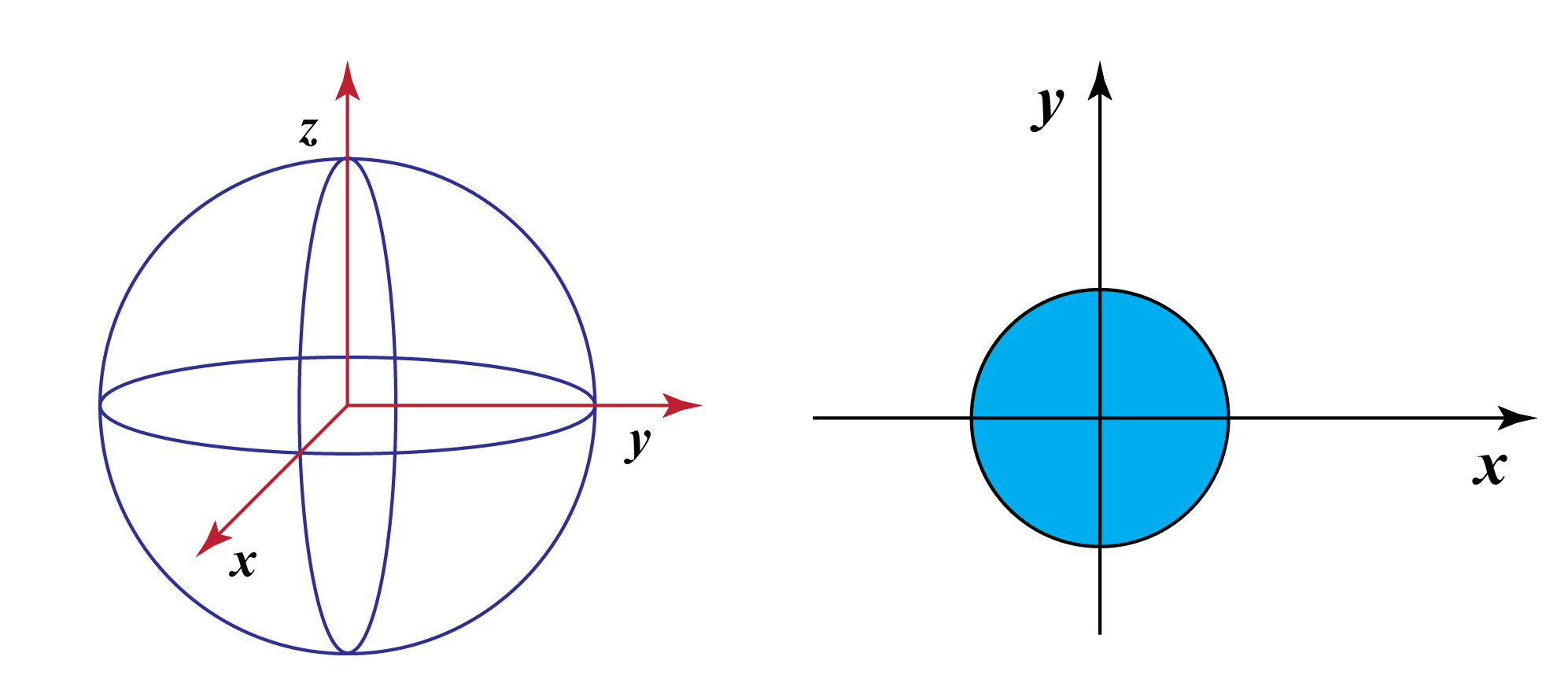3. A circle has a set of points at equal distance from the center of the plane whereas a sphere has a set of points at equal distance from the center of its axes.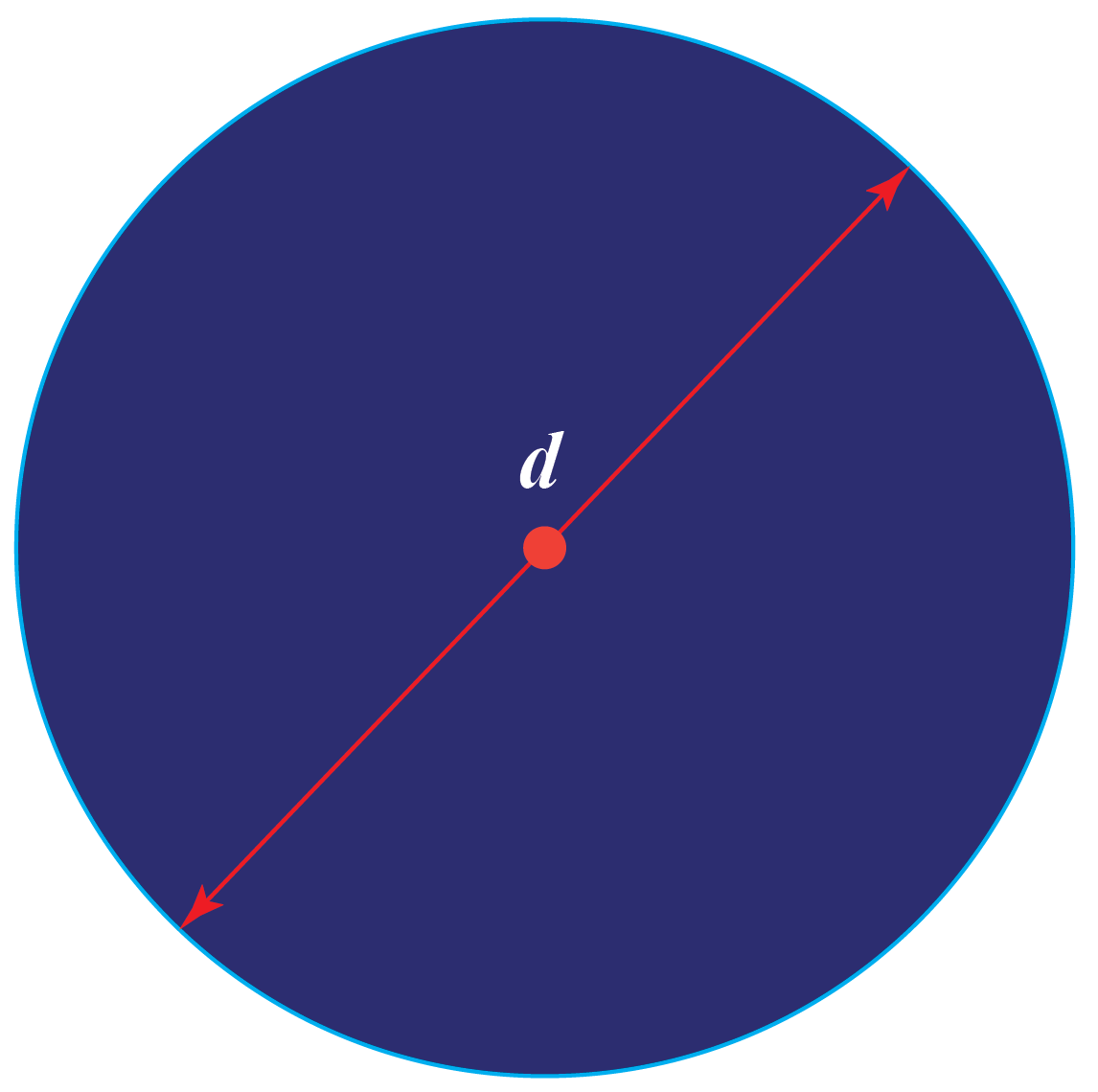4. Even though a circle and a sphere are round in shape, a sphere has some volume or depth.

## Solved Examples

 Example 1

Ruth says, "Oranges are spherical in shape."

Miranda says "Apples are spherical in shape."

Which fruit exactly resembles a sphere?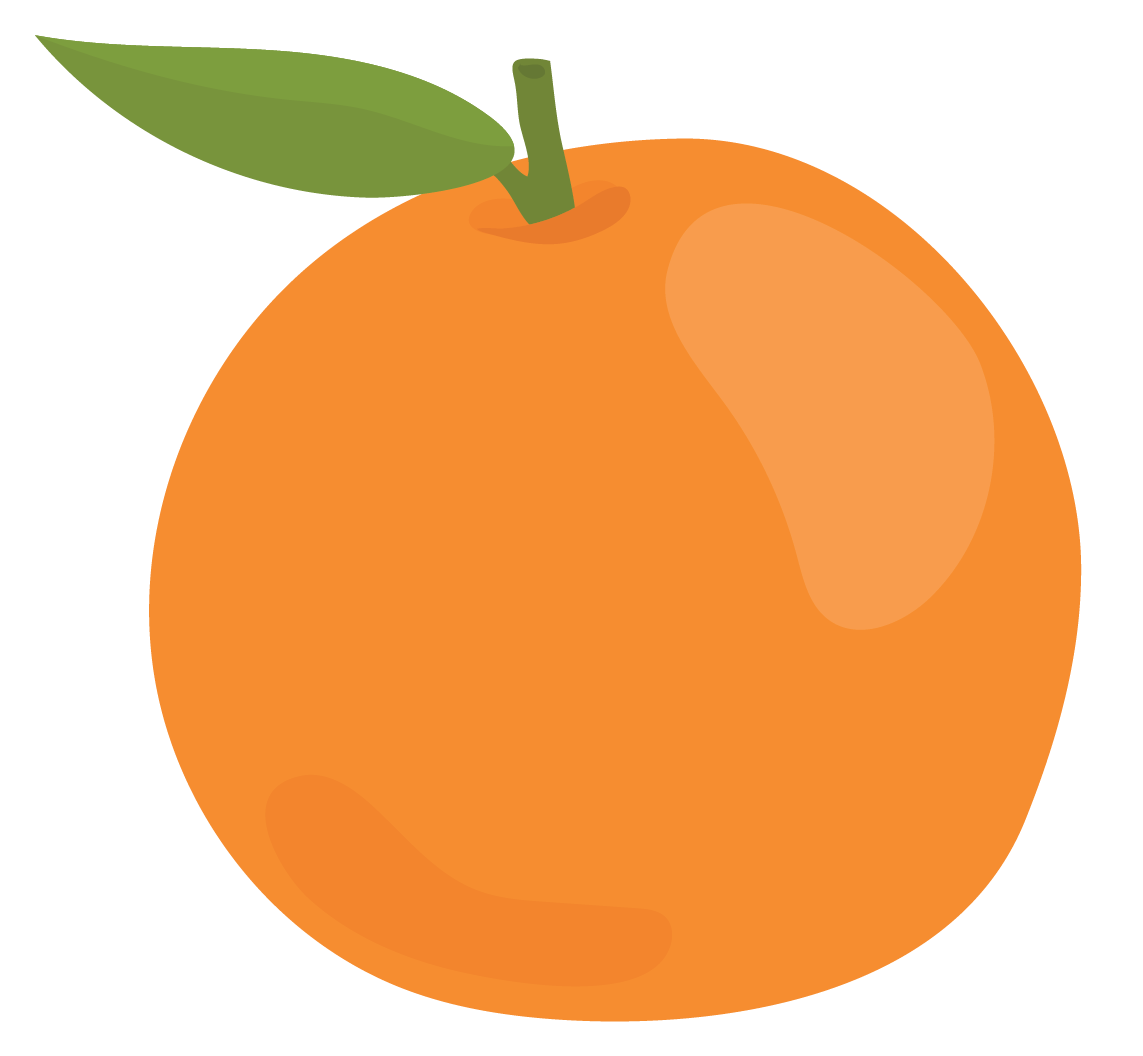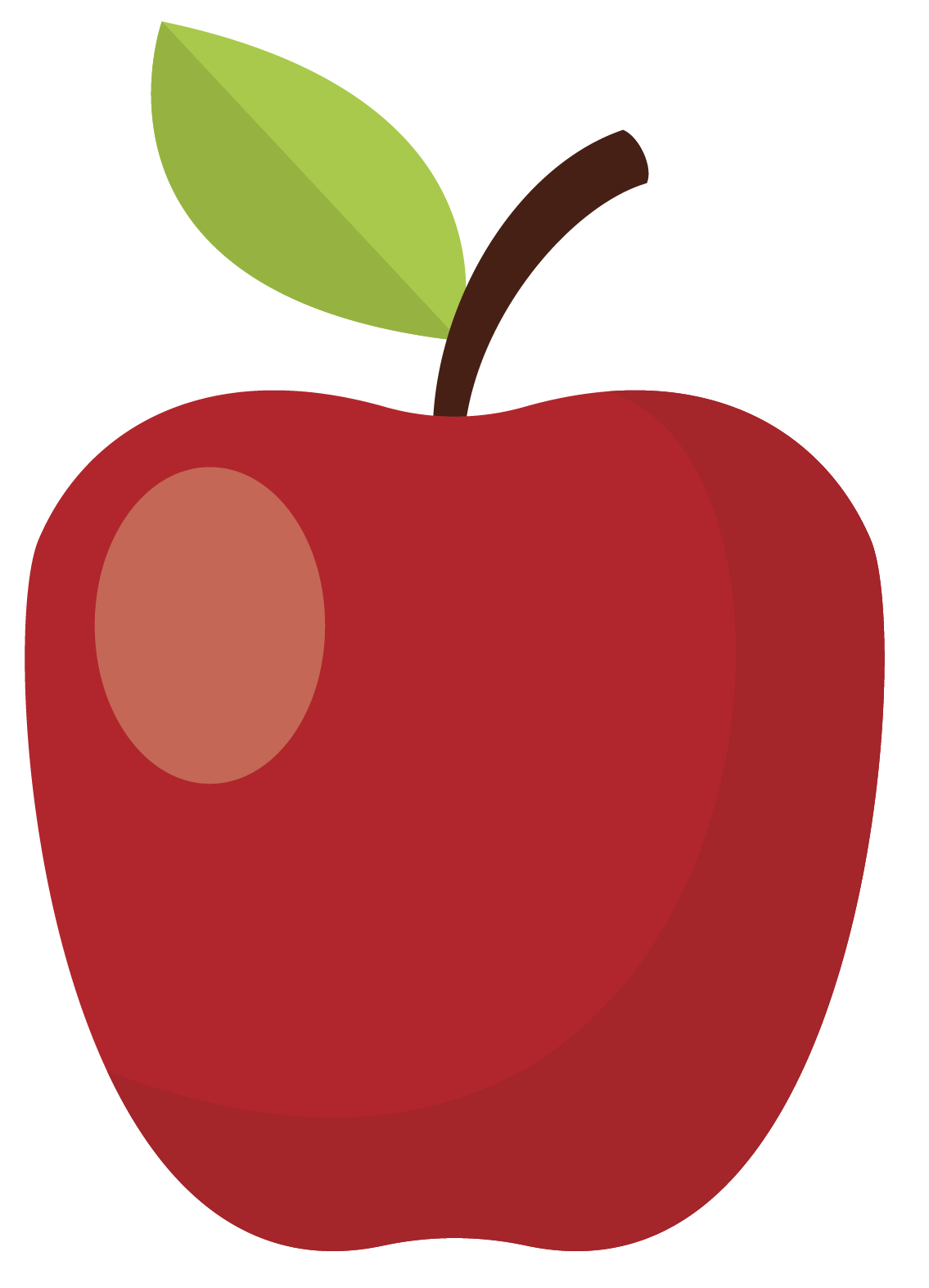Solution

A sphere is a three-dimensional figure, which is round in shape.An orange resembles a sphere; an apple is not exactly round.

 $$\therefore$$ An orange is spherical in shape.
 Example 2

Anna admires a hot-air balloon flying in the sky. Take $$\pi = \dfrac {22}{7}$$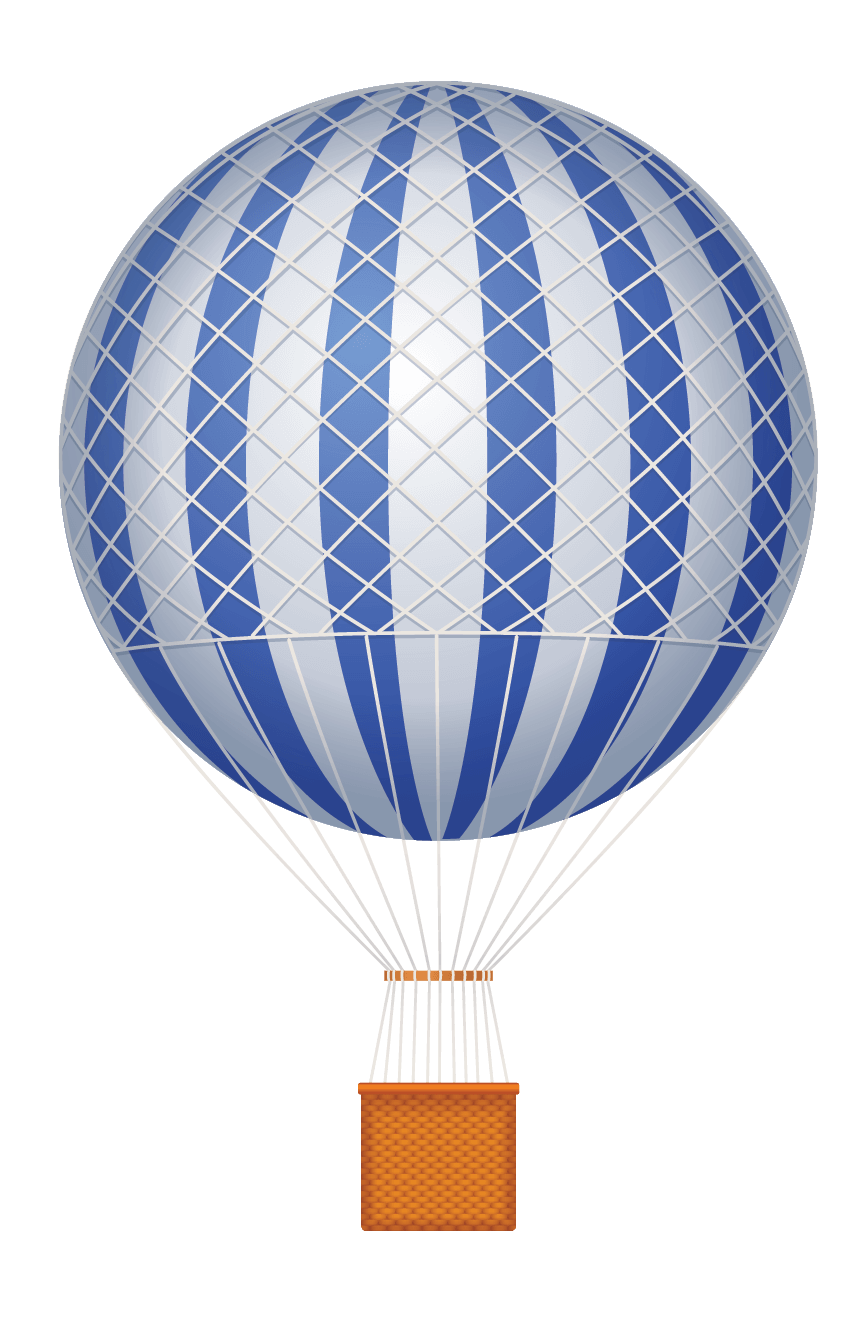The radius of the hot-air balloon is 7 feet.

Help Anna to find its volume.

Solution

The hot-air balloon is in the form of a sphere.

Sphere volume is obtained by the following formula:

\begin{align*}\text{Volume of a Sphere: V} &= \dfrac{4}{3}\pi r^{3}\\ \text V &= \frac{4}{3} \times \dfrac{22}{7} \times 7^{3}\end{align*}

 $$\therefore$$ The volume of the hot-air balloon is  $$\approx$$ 1437.33 cubic feet.
 Example 3

Edwin plans to decorate his Christmas tree with some colorful Christmas balls.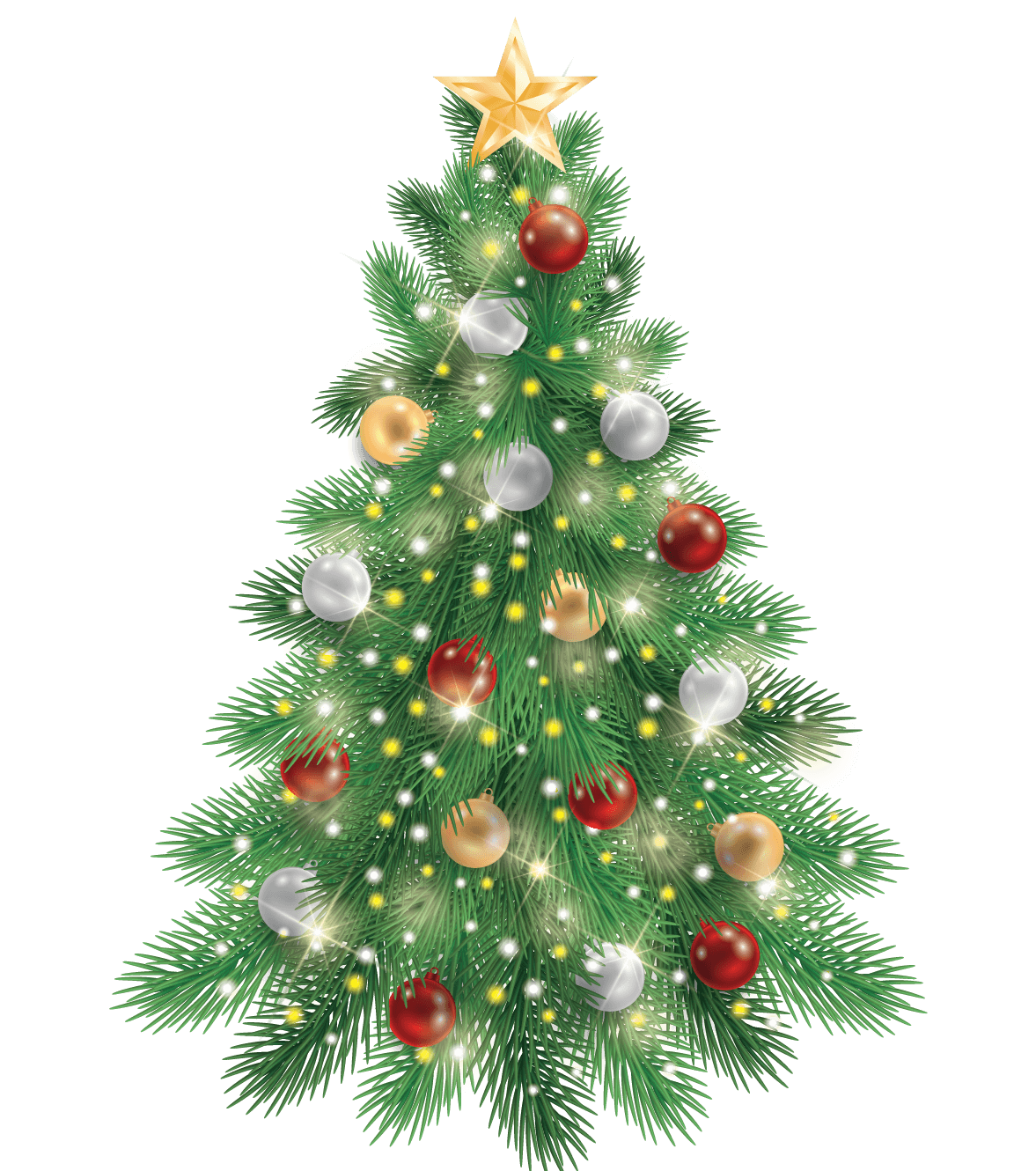He already has a Christmas ball with an area of 5 sq. inches.

Help Edwin to find the radius of a ball to buy more balls with the same radius.

Solution

The surface area of a Christmas ball is 5 sq. inches.

Since the ball is in a sphere shape, we will use its surface area formula.

\begin{align*}\text{Surface Area of a Sphere: A} &= 4\pi r^{2}\\ 5 &= 4 \times 3.14 \times r^{2}\\ r^{2} &= 0.3980\end{align*}

 $$\therefore$$ The radius of the Christmas ball is 0.63 inches.

## Interactive Questions

Here are a few activities for you to practice. Select/Type your answer and click the "Check Answer" button to see the result.Challenging Question
• Imagine that you have a melon whose volume is $$\begin{equation}\frac{4}{3}\pi r^{3}\end{equation}$$. Now you cut the melon horizontally into two equal halves. What will be the volume of one half of the melon?

## Let's Summarize

We hope you enjoyed learning about the sphere with the simulations and practice questions. Now you will be able to easily solve problems on the sphere volume formula, sphere surface area, and the fact that a sphere is three-dimensional.

At Cuemath, our team of math experts is dedicated to making learning fun for our favorite readers, the students!

Through an interactive and engaging learning-teaching-learning approach, the teachers explore all angles of a topic.

Be it worksheets, online classes, doubt sessions, or any other form of relation, it’s the logical thinking and smart learning approach that we at Cuemath believe in.

## 1. Does a sphere have a face?

A face is referred to as a flat or curved surface on a three-dimensional object.

For instance, a cube has 6 faces. Thereby, a sphere has only one face which is a curved surface.

## 2. Does a perfect sphere exist?

Yes! A perfect sphere exists in nature such as air bubbles, water drops, etc.

## 3. What is the difference between a circle and a sphere?

a. A circle is a two-dimensional figure whereas a sphere is a three-dimensional object.

b. A circle is extended in the x-axis and the y-axis whereas a sphere is extended in three directions (x-axis, y-axis, and z-axis).

c. A circle has a surface area only but a sphere has surface area and volume.

## 4. Is a sphere three-dimensional?

Yes. A sphere is a three-dimensional object that occupies the x-axis, y-axis, and z-axis in space.

More Important Topics
Numbers
Algebra
Geometry
Measurement
Money
Data
Trigonometry
Calculus